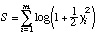# Manual Page for Command >>> CAUCHY

Syntax
>>> CAUCHY

Parent Command
>> OPTION

Subcommand
-

Description
This command selects an objective function that corresponds to a Cauchy or Lorentzian distribution, i.e., the probability density function of the residuals r reads:This distribution exhibits more extensive tails compared to the normal distribution, and leads therefore to a more robust estimation if outliers are present. The objective function to be minimized is given by the following equation:withThis objective function can bE minimized using the standard Levenberg-Marquardt algorithm which is designed for a quadratic objective function. The objective function can be reasonably well approximated by a quadratic function, so that the Levenberg-Marquardt algorithm is usually quite efficient.

Example
> COMPUTATION
>> OPTION
>>> assume measurement errors follow a CAUCHY distribution
<<<
<<